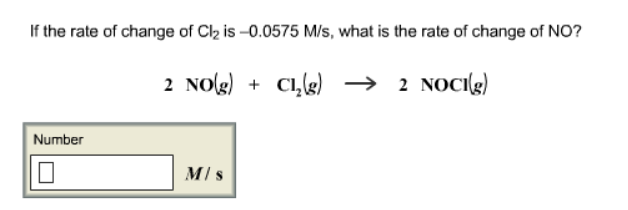# Problem: If the rate of change of Cl2 is -0.0575 M/s, what is the rate of change of NO? 2 NO (g) + Cl (g) → 2NOCl (g)

###### FREE Expert Solution
99% (424 ratings)###### Problem Details

If the rate of change of Cl2 is -0.0575 M/s, what is the rate of change of NO?

2 NO (g) + Cl (g) → 2NOCl (g)